Courses

# Test: Graphs & Hashing- 3

## 10 Questions MCQ Test Question Bank for GATE Computer Science Engineering | Test: Graphs & Hashing- 3

Description
This mock test of Test: Graphs & Hashing- 3 for Computer Science Engineering (CSE) helps you for every Computer Science Engineering (CSE) entrance exam. This contains 10 Multiple Choice Questions for Computer Science Engineering (CSE) Test: Graphs & Hashing- 3 (mcq) to study with solutions a complete question bank. The solved questions answers in this Test: Graphs & Hashing- 3 quiz give you a good mix of easy questions and tough questions. Computer Science Engineering (CSE) students definitely take this Test: Graphs & Hashing- 3 exercise for a better result in the exam. You can find other Test: Graphs & Hashing- 3 extra questions, long questions & short questions for Computer Science Engineering (CSE) on EduRev as well by searching above.
QUESTION: 1

### Let M be a 3 x3, adjacency matrix corresponding to a given graph of three nodes labeled 1,2, 3. If entry (1,3) in M3 is 2, then the graph could be

Solution:

If the (1, 3) entry in M3 is 2, it means there are 2 paths of length 3, connecting nodes 1 and 3. If you see the graph in option (a), there are 2 paths connecting 1 and 3,(1 → 2 → 3 → 3 and 1 → 3 → 3 → 3).

QUESTION: 2

### Consider the graph in figure: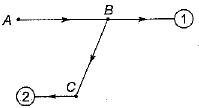What should be the labels of nodes marked 1 and 2 if the breadth first traversal yields the list a b c d e?

Solution:

In Breadth first traversal the nodes are searched level by level. Starting with vertex A, the only next choice is B. Then C, then 1 and lastly 2. Comparing with ABCDE.

QUESTION: 3

### Consider the graph in: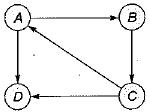Which of the following is a valid strong component?

Solution:

Strong component of a given graph is the maximal set of vertices such that for any two vertices i, j in the set, there is a path connecting i to j.
Obviously vertex ‘d' can’t be in the maximal set (as no vertex can be reached starting from vertex d).

QUESTION: 4

Consider the undirected weighted graph in figure. The minimum cost spanning tree for this graph has the cost.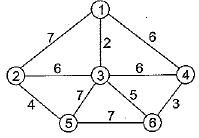Solution:

Using the Kruskal’s algorithm: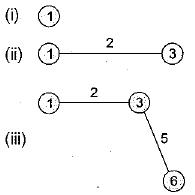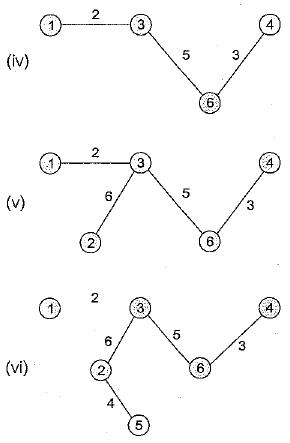∴ Minimum cost is 20.

QUESTION: 5

The number of binary trees with 3 nodes which when traversed in post-order gives the sequence A, B, C is

Solution:

Number of binary tree with 3 nodes with post order A, B, C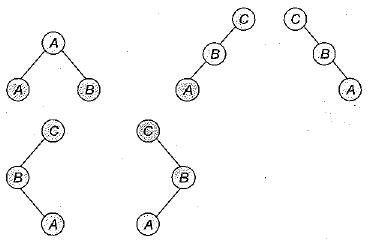So there are 5 binary tree possible.

QUESTION: 6

The minimum number of colors needed to color a graph having n (> 3) vertices and 2 edges is

Solution:

As we observe that the graph is disconnected
n > 3 (number of vertices)
e = 2 (number of edges)
For example : n = 4
for this only 2 colors are sufficient.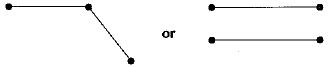QUESTION: 7

Which one of the following statements is false?

Solution:

Breadth first search can be used to find connected components of a graph.

QUESTION: 8

The number of edges in a regular graph of degree d and n vertices is

Solution:

In a regular graph, all the vertices will be of the same degree. Total degrees of all the vertices is nd. Each edge will be increasing the total degree by 2. So, totally nd/2 edges.

QUESTION: 9

The number of binary relations on a set with n elements is

Solution: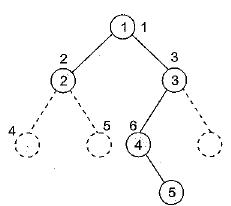Number of elements in set = n
Number of possible binary relations = n2
Number of possible binary relations of the set = 2n2.

QUESTION: 10

How many real links are required to store a sparse matrix of 10 rows, 10 columns, and 15 non-zero entries, (Pick up the closest answer)

Solution:

Matrix of 10 rows and 10 columns can have m axim um 1 0 x 1 0 = 100 entries. S ince only 15 non-zero entries are present, so number of real link will be 15 only.
Assuming undirected graph.